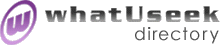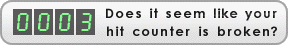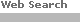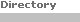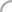SearchThe Directory The Webfor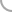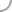EducationNew! Submit a site

whatUseek Directory Site Listings:

Math Forum Quick Reference - resources for teaching all levels of math K-12

Math Forum: Search mam-list - April is Math Awareness Month (MAM). For more information about MAM, consult the postings of the MAM-list.

Math Is Power - Targeted to middle and high school students and their families, site includes math games, a resource room and national resource registry.

Math and Education - Chris Smith - Homework, assignments, and tests for mathematic classes at Chattahoochee Tech (CTI) and Georgia Perimeter College (GPC). Also focuses on education courses from Georgia State (GSU).

Math-abundance - Tutorial

Math.com - homework help, tutoring, formulas.

MathCorner - A math site where visitors can test their math skills and do math research. Also, visitors can by math supplies like: books, calculators, and software.

MathMagic - K-12 telecommunications project motivates students to use computer technology while increasing problem-solving strategies and communications skills. MathMagic posts challenges in four categories (k-3, 4-6, 7-9 and 10-12) to trigger each registered team to pair up with another team and engage in a problem-solving dialog.

MathSphere - For teachers and parents to download practice worksheets and tests to improve children's achievement. Competitions, children's pages and children's contributions.

MathWorld Interactive - Participating students from all over the world work on open-ended word problems called MathWorld Interactive Challenges. During the cycle, students discuss, share ideas, meet each other, and ask questions on the he MathWorld Interactive Message Boards.

Mathematical Quotations Server - Which famous mathematician said, "The whole is more than the sum of its parts?"(Answer: Aristotle). The answer lies at the Mathematical Quotations Server, courtesy of Furman University.

Mathematics Experiences Through Image Processing (METIP) - Materials that promote digital image processing as a means of motivating K-12 students in mathematics and as a means of teaching mathematical concepts.

Mathematics Help Central - Devoted to helping the searching math student, this site includes topics concerning all aspects of mathematics. Also includes class notes.

Mathematics Learning Support Centre - A pamphlet of mathematical facts and formulas.

Mathematics Reference - A collection of math related tables, facts and formulas.

Mathematics Reference - A collection of math related tables, facts and formulas.

Mathematics Reference: Math Tables, Facts and Formulas - A mathematics reference collection of "K through 14" math tables, facts, definitions, formulas and explanations ... from general math through college calculus.

Mathematics, Statistics and Operational Research - Information, resources and links to learning and teaching.

Mathguide - A mathematics-related, one-stop website for interactive lessons, puzzles, educational issues, terminology, help, projects and lessons.

MathsSkills - Mathskills will provide information to the mathematical community through its Web Homepage, which will include regular newsletters, survey data, a discussion group and conference details, as well as gradually establishing reference material such as expert lists, exemplars of good practice, case studies and other support material.

[ 1 2 3 4 5 6 ]Help build the largest human-edited directory on the web.About   Help   Content Filter   Terms   Privacy Policy© 2018 whatUseek